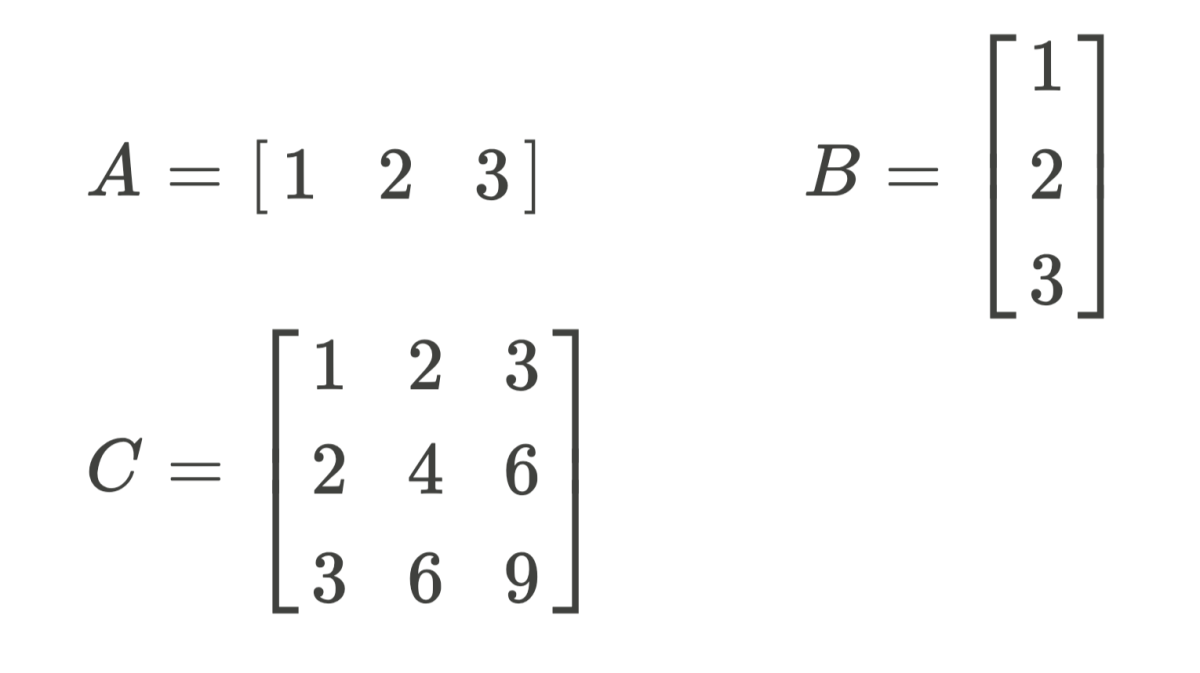# An Introduction to Linear Algebra

Davinder Singh is a Polymath. He loves reading, learning and writing technical cookbooks about new technologies.

## Content

• What is Linear Algebra?
• Vectors, Matrices and Linear Maps
• Column and Row Vectors
• Algebra of Vectors
• Final Note

## What is Linear Algebra?

Linear algebra is the study of Linear Systems. A Linear System is a system whose state is described in terms of lines in n-dimensions. Every line forms a vector, and the set of these vectors form Vector Space. Dimension is a finite entity, the value of which depends upon the number of variables a system has.

Vectors can be equated with others via Linear Equations. These equations help us in understanding the system and discover its properties (invertible, singular etc.). They convert gracefully to matrices. Matrices help in shortening the work because now we can manipulate an equation in n-dimensions as a matrix of n-dimensions.

## Vectors, Matrices and Linear Maps

Vector is nothing but a list of objects. Now these objects can be anything (I am oversimplifying things here) such as Real, Natural or Complex numbers. Vectors also come in two different shapes: Columns and Row vectors. The shape of a vector holds special importance in vector algebra.

A Vector Space is a collection of vectors. We differentiate between vector spaces by the dimensions they have. For example, a two-dimensional vector space has vectors that contain two components, viz. x-component and y-component, whereas a three-dimensional vector space has three: x-, y- and z-components.

Matrices are formed by combining multiple (row or column) vectors together. Matrices help us deal with big vectors in an organized manner. Linear Equations translate effortlessly to matrices, and then we apply matrix magic to find components. In another article, I will completely focus on matrices and various operations that can be performed over them.

Linear Maps are functions that relate one vector space to that of another. They help us in comparing an unknown vector space with a known one. Also, Linear Maps transform vectors belonging to one coordinate system to another. They also translate, rotate and skew vectors. These operations all are represented as Matrices.

## Columns and Row Vectors

Vectors come in two forms, which are Column and Row vectors. A column vector is like a pole of numbers (vertical) whereas a Row vector is a set of numbers (horizontal). These shapes are analogous to the negative sign used in scalars (ordinary numbers) to represent the fact that one is opposite to another in terms of direction but has the same magnitude (-7 and 7, for example, are reflections of each other on the number line).A is row vector, B is column vector while C is matrixAuthor

## Algebra of Vectors

Manipulation of vectors is no different from working with scalar values, although some things have to be taken care of, such as the shape of vectors and the fact that we are working on multiple numbers at once. Three basic operations that can be applied to vectors are addition, multiplication by scalars and multiplication by another vector.

The addition of two vectors can only be performed when both vectors have the same shape and size (dimension). It is computed by adding individual components together. The resultant vector has a similar shape and size.

Scroll to Continue

Subtraction is performed similarly—the only difference is we subtract instead of adding.

Note: Addition (or Subtraction) cannot be performed between row and column vectors and vice-versa. Also, we cannot perform addition between 2-dimensional and 3-dimensional vectors.

2. Multiplication by Scalar

This operation is to scale-up or scale-down a given vector. Imagine a 2-d line with x, y coordinates as (2,1) and we want to double the length of this line without altering its direction. So, how do we do it? By multiplying both components by 2, that is 2*(2,1) = (4,2). This is called multiplication by scalar. Scalar Multiplication can be performed without caring about the shape and size of the vector. The resultant vector is of similar shape and size.

3. Dot Product

Dot Product is one of the most exciting and one of the most misunderstood operations of Linear Algebra. By performing dot-product, we are actually doing both addition and multiplication. Unlike the product of scalars, dot-product is not commutative, and also we cannot always perform it. Application of it alters both the direction and length of the vector.

Dot Product can only be applied between vectors/matrices v1(n, m) and v2(x, y) when m is equal to x. The resulting vector/matrix is of size v3(n, y).

The intuition behind dot-product is that it's analogous to function composition, which is compositing two functions to have the combined effect of both. For example, consider the function F(x) = x+2 and G(x) = x*x.

Let's combine them both to FoG (x) = (x*x)+2 or GoF(x) = (x+2)*(x+2), since FoG and GoF both have different compositions of functions, like matrix dot-product is not commutative. In the case of matrices, we are compositing Linear Maps together: Rotate by 45 degrees and then scale by 3.